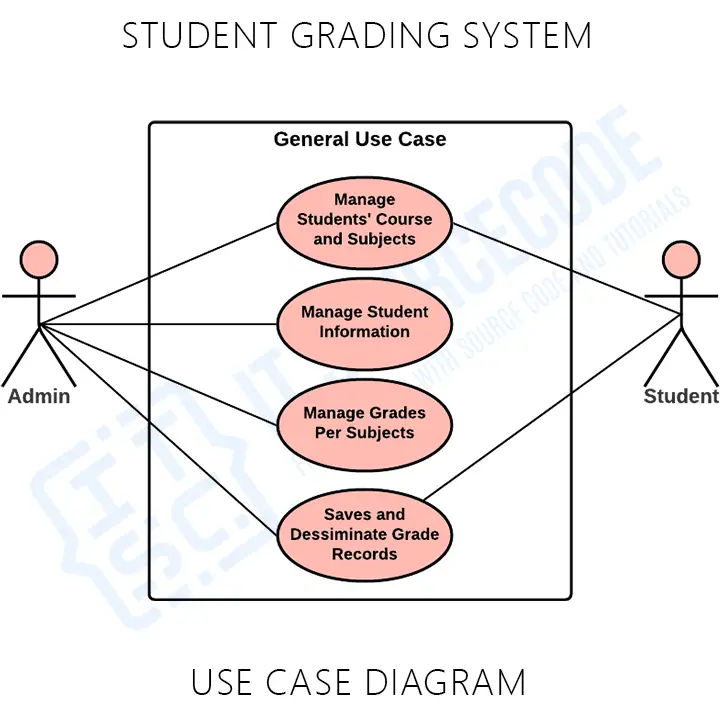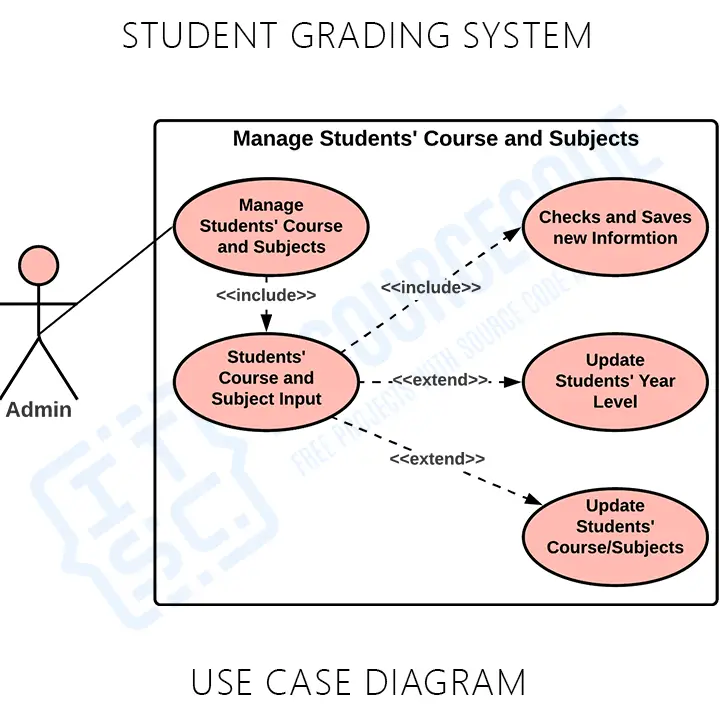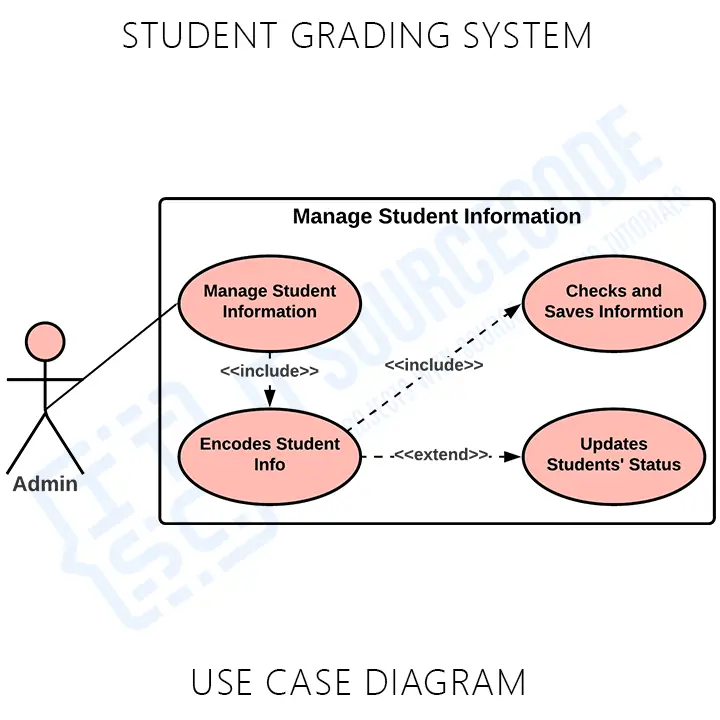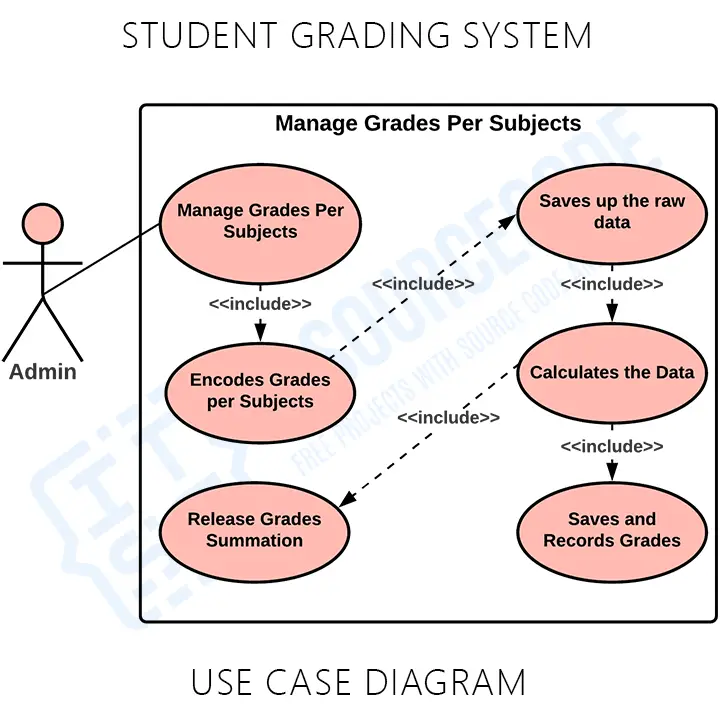# Student Grading System Use Case Diagram

## Student Grading System Project Use Case Diagram using Include and Extend (UML)

Hi there Itsourcecoders! Today I will give you ideas about the Student Grading System Use Case Diagram and Description for 2021. This article discusses the meaning of the Student Grading System project UML as well as its use case diagram using include and extend. By the end of the discussion you will find the downloadable use case diagram PDF file for Student Grading System that can be your basis.

To start with, let us know what does a use case mean.

A use case diagram is a visual representation of how a user might interact with a program. A use case diagram depicts the system’s numerous use cases and different sorts of users. The circles or ellipses are used to depict the use cases.

### Student Grading System Project Use Case Diagram Potential Features:

The features of the system depends on the users of it.

• Monitor and Manage Student Courses and Subjects
• Manage Student Information

By creating the use case of the Student Grading System, you must determine first the possible features to identify the flow of the system. After that you can now create the blueprint or core of the system function.

## Student Grading System Project Use Case Diagram

Here are the diagrams with discussion of Student Grading System Project Use Case Diagram using Include and Extend 2021.

1. General Use Case

Now I present to you the general use case of the Student Grading System. This diagram shows the general processes or function that the system could do that is based on the data given by the students and then fed to the system. The system then will calculate the grades given, records it and given to the students by either the grading admin or teachers.General use case is the most common application of a use case diagram. The use case diagrams depict the system’s main components as well as the flow of information between them.

With the help of this general use case, the programmer will have the basis on what could be put into consideration in creating the student grading system.

2. Monitor and Manage Student Courses and Subjects

This is where the admin or the main user of the system could control the information of the courses and listed down its required or pre requisite subjects. These information were encoded to the system to be the basis of the admin as they calculate the given data by the students. The system then will monitor its details to validate it and then saves the data.3. Manage Student Information

This is the process where the admin will have to manage every student information regarding with what year level they are in, on what course and their subjects taken. These information were the basis for the collecting of data up to calculating and giving the summation of their grades.This process explains about how the admin or users handles the data given by the teachers to their students by their subjects taken. by this process, the teachers will not have to manually solve their grades but to just fed their outputs or raw grades into the system and then the system will do the rest.## Additional Knowledge to Student Grading System Use Case Diagram and Description

The objective of a use case diagram is to show the interactions of numerous items called actors with the use case and to capture fundamental functionalities of a system. As you see through the diagrams, there are the use cases involved to define the core functions of a system. These processes were expected by the users to be connected to produce a certain output. Being a programmer, this could be an important role that the Student Grading should have.